# Gauss's Law

Table of Content

## 1. Introduction

• Gauss's law was suggested by Karl Frederich Gauss(1777-1855) who was German scientist and mathematician.
• Gauss's law is basically the relation between the charge distribution producing the electrostatic field to the behavior of electrostatic field in space.
• Gauss's law is based on the fact that flux through any closed surface is a measure of total amount of charge inside that surface and any charge outside that surface would not contribute anything to the total flux.
• Before we look further to study Gauss's law in detail let's study electric field due to continuous charge distributions.

## 2. Electric field due to continous charge distributions

• So far as in the previous chapter we have discussed force and field due to discrete charges.
• We now assume that charges on a surface are located very close togather so that such a system of charges can be assumed to have continous distribution of charges.
• In a system of closely spaced charges, total charge could be continously distributed along some line, over a surface or throughout a volume.
• First divide the continous charge distribution into small elements containing Δq amount of charge as shown in fig 1

•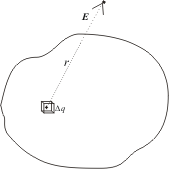• Electric field at point A due to element carrying charge Δq is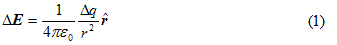where r is the distance of element under consideration from point A and rˆis the unit vector in the direction from charge element towards point A.
• Total electric field at point A due to all such charge elements in charge distribution is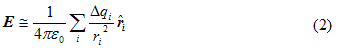where index i refers to the ith charge element in the entire charge distribution.
• Since the charge is distributed continuously over some region , the sum becomes integral. Hence total field at A within the limit Δq→0 is,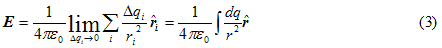and integration is done over the entire charge distribution.
• If a charge q is uniformly distributed along a line of length L, the linear charge density λ is defined by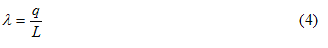and the unit of λ is Coulumb/meter(C/m).
• For charge distributed non-uniformly over a line, linear charge density is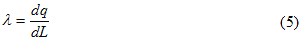where dQ is the amount of charge in a small length element dL.
• For a charge Q uniformly distributed over a surface of area A, the surface charge density σ is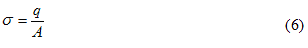and unit of surface charge density is C/m2. For non uniform charge distributed over a surface charge density is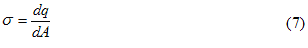where dA is a small area element of charge dQ.
• Similarly for uniform charge distributions volume charge density is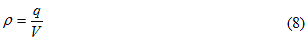and for non uniform distribution of charges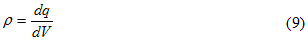and unit of volume charge distribution is C/m3.

## 3. Gauss's Law

• We already know about electric field lines and electric flux. Electric flux through a closed surface S is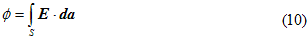which is the number of field lines passing through surface S.
• Statement of Gauss's Law
“ELectric flux through any surface enclosing charge is equal to q/ε0 , where q is the net charge enclosed by the surface”
mathematically,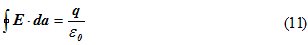where qenc is the net charge enclosed by the surface and E is the total electric field at each point on the surface under consideration.
• It is the net charge enclosed in the surface that matters in Gauss's law but the total flux of electric field Edepends also on the surface choosen not merely on the charge enclosed.
• So if you have information about distribution of electric charge inside the surface you can find electric flux through that surface using Gauss's Law.
• Again if you have information regarding electric fluxthrough any closed surface then total charge enclosed by that surface can also be easily calculated using Gauss's Law.
• Surface on which Gauss's Law is applied is known as Gaussian surface which need not be a real surface.
• Gaussian surface can be an imaginary geometrical surface which might be empty space or it could be partially or fully embedded in a solid body.
• Again consider equation 11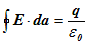In left hand side of above equation E·da is scalar product of two vectors namely electric field vector E and area vector da. Area vector da is defined as the vector of magnitude |da| whose direction is that of outward normal to area element da. So,da=nˆda where nˆ is unit vector along outward normal to da.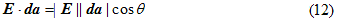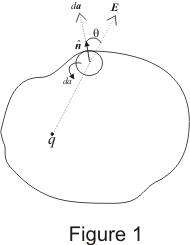From above discussion we can conclude that,
(1) If both E and surface area da at each points are perpendicular to each other and has same magnitude at all points of the surface then vector E has same direction as that of area vector as shown below in the figure.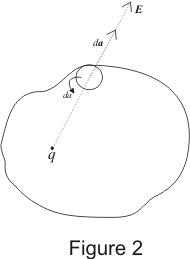since E is perpendicular to the surface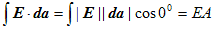(2) If E is parallel to the surface as shown below in the figure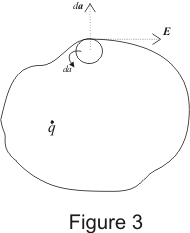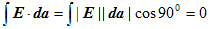and integral is also zero for zero value of electric field vector E at all points on the surface.

## Applications of Gauss's Law

Gauss law has many applications in Electrostatics. Gauss's law can be used to solve complex problems on electric field. We can easily solve the complex electrostatic problems involving unique symmetries like cylindrical, spherical or planar symmetry with the help of Gauss's law.Lets take a look at some of the important and common one

### (A) Derivation of Coulumb's Law

• Coulumb's law can be derived from Gauss's law.
• Consider electric field of a single isolated positive charge of magnitude q as shown below in the figure.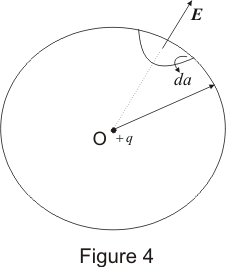• Field of a positive charge is in radially outward direction everywhere and magnitude of electric field intensity is same for all points at a distance r from the charge.
• We can assume Gaussian surface to be a sphere of radius r enclosing the charge q.
• From Gauss's law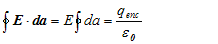since E is constant at all points on the surface therefore,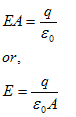surface area of the sphere is A=4πr2
thus,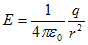• Now force acting on point charge q' at distance r from point charge q is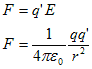This is nothing but the mathematical statement of Coulomb's law.

### (B) Electric field due to line charge

• Consider a long thin uniformly charged wire and we have to find the electric field intensity due to the wire at any point at perpandicular distance from the wire.
• If the wire is very long and we are at point far away from both its ends then field lines outside the wire are radial and would lie on a plane perpandicular to the wire.
• Electric field intensity have same magnitude at all points which are at same distance from the line charge.
• We can assume Gaussian surface to be a right circular cylinder of radius r and length l with its ends perpandicular to the wire as shown below in the figure.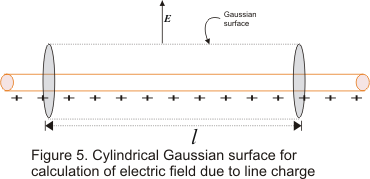• λ is the charge per unit length on the wire. Direction of E is perpendicular to the wire and components of E normal to end faces of cylinder makes no contribution to electric flux. Thus from Gauss's law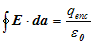• Now consider left hand side of Gauss's law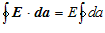Since at all points on the curved surface E is constant. Surface area of cylinder of radius r and length l is A=2πrl therefore,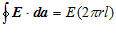• Charge enclosed in cylinder is q=linear charge density x length l of cylinder,
or, q=λl
From Gauss's law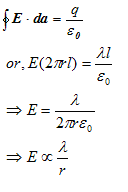Thus electric field intensity of a long positively charged wire does not depends on length of the wire but on the radial distance r of points from the wire.

### (C) Electric field due to charged solid sphere

• We'll now apply Gauss's law to find the field outside uniformly charged solid sphere of radius R and total charge q.
• In this case Gaussian surface would be a sphere of radius r>R concentric with the charged solid sphere shown below in the figure.From Gauss's lawwhere q is the charge enclosed.
• Charge is distributed uniformly over the surface of the sphere. Symmetry allows us to extract E out of the integral sign as magnitude of electric field intensity is same for all points at distance r>R.
• Since electric field points radially outwards we have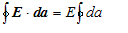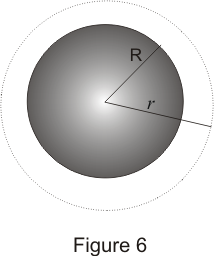also as discussed magnitude of E is constant over Gaussian surface so,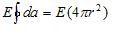where 4πr2 is the surface area of the sphere.
Again from Gauss's law we have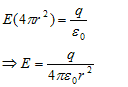THus we see that magnitude of field outside the sphere is exactly the same as it would have been as if all the charge were concentrated at its center.

### (D) Electric field due to an infinite plane sheet of charge

• Consider a thin infinite plane sheet of charge having surface charge density σ(charge per unit area).
• We have to find the electric field intensity due to this sheet at ant point which is distance r away from the sheet.
• We can draw a rectangular gaussian pillbox extending equal distance above and below the plane as shown below in the figure.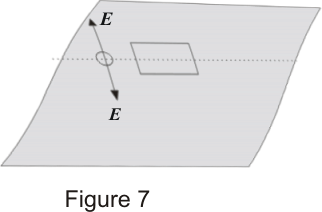• By symmetry we find that Eon either side of sheet must be perpendicular to the plane of the sheet, having same magnitude at all points equidistant from the sheet.
• No field lines crosses the side walls of the Gaussian pillbox i.e., component of E normal to walls of pillbox is zero.
• We now apply Gauss's law to this surfacein this case charge enclosed is
q=σA
where A is the area of end face of Gaussian pillbox.
• E points in the direction away from the plane i.e., E points upwards for points above the plane and downwards for points below the plane. Thus for top and bottom surfaces,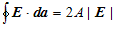thus
2A|E|=σA/ε0
or,
|E|=σ/2ε0
Here one important thing to note is that magnitude of electric field at any point is independent of the sheet and does not decrease inversely with the square of the distance. Thus electric field due to an infinite plane sheet of charge does not falls of at all.